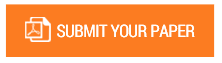Moment based Estimation for the Shape Parameters, Effect it on Some Probability Statistical Distributions Using the Moment Method and Maximum Likelihood Method

Authors : Hassan Tawakol A. Fadol

Volume/Issue : Volume 5 - 2020, Issue 6 - June

Scribd : https://bit.ly/3gK52wt

DOI : 10.38124/IJISRT20JUN551

The purpose of this paper was to identify the values of the parameters of the shape of the binomial, bias one and natural distributions. Using the estimation method and maximum likelihood Method, the criterion of differentiation was used to estimate the shape parameter between the probability distributions and to arrive at the best estimate of the parameter of the shape when the sample sizes are small, medium, The problem was to find the best estimate of the characteristics of the society to be estimated so that they are close to the estimated average of the mean error squares and also the effect of the estimation method on estimating the shape parameter of the distributions at the sizes of different samples In the values of the different shape parameter, the descriptive and inductive method was selected in the analysis of the data by generating 1000 random numbers of different sizes using the simulation method through the MATLAB program. A number of results were reached, 10) to estimate the small shape parameter (0.3) for binomial distributions and Poisson and natural and they can use the Poisson distribution because it is the best among the distributions, and to estimate the parameter of figure (0.5), (0.7), (0.9) Because it is better for binomial binomial distributions, when the size of a sample (70) for a teacher estimate The small figure (0.3) of the binomial and boson distributions and natural distributions can be used for normal distribution because it is the best among the distributions.

Keywords : Shape parameter, parameters estimation, Binomial distribution, Random Numbers, The Moment Method, Maximum Likelihood Method.

#### CALL FOR PAPERS

Paper Submission Last Date
30 - June - 2023